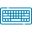# Riemann Sum Calculator## Table of Content

Feedback

By using the finite sum Riemann sum calculator displays the approximation of an integral for an area under the curve and also evaluates the sample points of a midpoint, trapezoid, left endpoint, and right endpoint.

## What does Riemann Sum Mean?

“The way of numeric integration that finds the approximate value of a definite integral which is an area under a curve for a given interval”.

It is the creation of German Mathematician B.Riemann (1826−1866)

## Types of Riemann Sum:

There are the following types of this integration method but the difference between them is the location of the point at which the function is determined to find the height of the rectangle. Riemann Sum Calculator finds the approximated value of the area of the curve with steps

### 1. Left Riemann Sum:

The left endpoint Riemann sum is also known as the left endpoint approximation. It uses the left subinterval for height evaluation of the approximating rectangle. In this type, the height of each rectangle will be given by f(a+iΔx) and the base equals the Δx.

• Left Riemann Sum Formula:

∑ni = 1f (xi) Δx

If f is increasing then their minimum will always be on the left side so we say the left sum is underestimated.

### 2. Right Riemann Sum:

Right hand Riemann sum is the method that accumulates the area of a rectangle to approximate the area under a curve. In this type, the height of each rectangle will be given by f(a+iΔx) and the base equals the Δx.

• Right Riemann Sum Formula:

∑ni = 1f (xi + 1) Δx

If f is increasing its maximum will always be on the right side so we say that the right sum is always an overestimated.

### 3. Midpoint Riemann Sum:

Midpoint Riemann sum touches the curve at both of its top vertices. In this specific method, the height of each rectangle is equal to the value of the function at its midpoint

• Midpoint Riemann Sum Formula:

∑ni = 1f (xi + xx + 12) Δx

## Riemann Sum Equation:

Approximate the area of a curve using the Riemann sum calculator step-by-step or if you come for manual calculation then use the following Riemann sum formula.

$$S = \sum_{i=1}^n f(x^*_i) Δx$$

## Working of Riemann Sum Calculator:

The right endpoint approximation calculator finds the approximated value of the area of the curve by taking into service the below points:

Input:

• Put the values of the function that you have
• Insert the lower and upper limit
• Choose the type of Riemann sum
• Set the values of rectangles
• Tap the “Calculate”

Output:

See what the result will be if you put the above values in the Riemann sums calculator.

• A standard form of input equation with limits
• Complete steps of whole calculations

## References:

From the source Wikipedia: Riemann sum, Types of Riemann sums, Riemann summation methods, Connection with integration.

From the source Libretext: Riemann Sums, Using the Left Hand, Right Hand and Midpoint Rules, Summation Notation, properties of summation notation.

From the source brilliant.org: Riemann Sums, Relationship to Definite Integral, Application, and Examples, Definite Integral as a Limit of a Sum.### Alan Walker

Studies mathematics sciences, and Technology. Tech geek and a content writer. Wikipedia addict who wants to know everything. Loves traveling, nature, reading. Math and Technology have done their part, and now it's the time for us to get benefits.